PSAT Math : Basic Squaring / Square Roots

Example Questions

1 2 3 5 Next →

Example Question #1 : How To Square A Decimal

If all real values oflie between 0 and 1, which of the following is always greater than 1?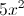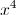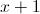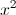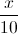Explanation:

Ifis greater than 0, then adding 1 towill make it greater than 1. Taking a number between 0 and 1 to a power results in a smaller number.

Example Question #2 : How To Square A Decimal

Evaluate:

0.082

0.00064

0.64

0.064

0.00064

0.0064

0.0064

Explanation:

0.08 * 0.08

First square 8:

8 * 8 = 64

Then move the decimal four places to the left:

0.0064

1 2 3 5 Next →